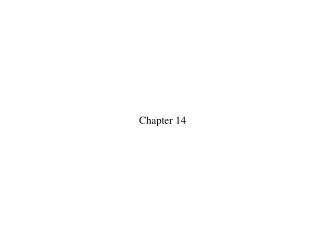DownloadDownload PresentationChapter 14

# Chapter 14

Télécharger la présentation## Chapter 14

- - - - - - - - - - - - - - - - - - - - - - - - - - - E N D - - - - - - - - - - - - - - - - - - - - - - - - - - -
##### Presentation Transcript

1. Chapter 14

2. An object moves with simple harmonic motion. If the amplitude and the period are both doubled, the object’s maximum speed is 1. quartered. 2. halved. 3. quadrupled. 4. doubled. 5. unchanged.

3. An object moves with simple harmonic motion. If the amplitude and the period are both doubled, the object’s maximum speed is 1. quartered. 2. halved. 3. quadrupled. 4. doubled. 5. unchanged.

4. The figure shows four oscillators at t = 0. Which one has the phase constant 1) a 2) b 3) c 4) d

5. The figure shows four oscillators at t = 0. Which one has the phase constant 1) a 2) b 3) c 4) d

6. Four springs have been compressed from their equilibrium position at x = 0 cm. When released, they will start to oscillate. Rank in order, from highest to lowest, the maximum speeds of the oscillators. 1. c > b > a = d 2. c > b > a > d 3. d > a > b > c 4. a = d > b > c 5. b > c > a = d

7. Four springs have been compressed from their equilibrium position at x = 0 cm. When released, they will start to oscillate. Rank in order, from highest to lowest, the maximum speeds of the oscillators. 1. c > b > a = d 2. c > b > a > d 3. d > a > b > c 4. a = d > b > c 5. b > c > a = d

8. This is the position graph of a mass on a spring. What can you say about the velocity and the force at the instant indicated by the dotted line? 1. Velocity is positive; force is to the right. 2. Velocity is negative; force is to the left. 3. Velocity is negative; force is to the right. 4. Velocity is zero; force is to the right. 5. Velocity is zero; force is to the left.

9. This is the position graph of a mass on a spring. What can you say about the velocity and the force at the instant indicated by the dotted line? 1. Velocity is positive; force is to the right. 2. Velocity is negative; force is to the left. 3. Velocity is negative; force is to the right. 4. Velocity is zero; force is to the right. 5. Velocity is zero; force is to the left.

10. One person swings on a swing and finds that the period is 3.0 s. Then a second person of equal mass joins him. With two people swinging, the period is 1. 1.5 s. 2. <3.0 s but not necessarily 1.5 s. 3. 3.0 s. 4. >3.0 s but not necessarily 6.0 s. 5. 6.0 s.

11. One person swings on a swing and finds that the period is 3.0 s. Then a second person of equal mass joins him. With two people swinging, the period is 1. 1.5 s. 2. <3.0 s but not necessarily 1.5 s. 3. 3.0 s. 4. >3.0 s but not necessarily 6.0 s. 5. 6.0 s.

12. Rank in order, from largest to smallest, the time constants of the decays shown in the figure. 1. 2. 3. 4. 5.

13. Rank in order, from largest to smallest, the time constants of the decays shown in the figure. 1. 2. 3. 4. 5.

15. What is the name of the quantity represented by the symbol 1. Angular momentum 2. Angular frequency 3. Phase constant 4. Uniform circular motion 5. Centripetal acceleration

16. What is the name of the quantity represented by the symbol 1. Angular momentum 2. Angular frequency 3. Phase constant 4. Uniform circular motion 5. Centripetal acceleration

17. What term is used to describe an oscillator that “runs down” and eventually stops? 1. Tired oscillator 2. Out of shape oscillator 3. Damped Oscillator 4. Resonant oscillator 5. Driven oscillator

18. What term is used to describe an oscillator that “runs down” and eventually stops? 1. Tired oscillator 2. Out of shape oscillator 3. Damped Oscillator 4. Resonant oscillator 5. Driven oscillator

19. The starting conditions of an oscillator are characterized by 1. the initial acceleration. 2. the phase constant. 3. the phase angle. 4. the frequency.

20. The starting conditions of an oscillator are characterized by 1. the initial acceleration. 2. the phase constant. 3. the phase angle. 4. the frequency.

21. Wavelength is • 1. the time in which an oscillation repeats itself. • 2. the distance in which an oscillation repeats itself. • 3. the distance from one end of an oscillation to the other. • 4. the maximum displacement of an oscillator. • 5. not discussed in Chapter 14.

22. Wavelength is • 1. the time in which an oscillation repeats itself. • 2. the distance in which an oscillation repeats itself. • 3. the distance from one end of an oscillation to the other. • 4. the maximum displacement of an oscillator. • 5. not discussed in Chapter 14.